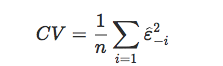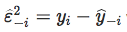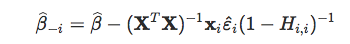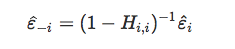{{announcement.body}}
{{announcement.title}}

# Econometric Models With (Pure) Random Features

DZone 's Guide to

# Econometric Models With (Pure) Random Features

· AI Zone ·
Free Resource

Comment (0)

Save
{{ articles.views | formatCount}} Views

For my lectures on applied linear models, I wanted to illustrate the fact that the R^2 is never a good measure of the goodness of the model since it's quite easy to improve it. Consider the following dataset:

n=100
df=data.frame(matrix(rnorm(n*n),n,n))
names(df)=c("Y",paste("X",1:99,sep=""))

...with one variable of interest y, and 99 features x_j. All of them being (by construction) independent. And we have 100 observations. Consider the regression on the first k features, and compute R_k^2 of that regression.

reg=function(k){
frm=paste("Y~",paste("X",1:k,collapse="+",sep=""))
model=lm(frm,data=df)
summary(model)$adj.r.squared} Let us see what's going on... plot(1:99,Vectorize(reg)(1:99))(Actually, it's not exactly what we have on the graph. We have the average obtained over 1,000 samples randomly generated, with 90 percent confidence bands). Observe that \mathbb{E}[R^2_k]=k/n, i.e. if we add some pure random noise, we keep increasing the R^2 (up to 1, actually). Good news, as we've seen in the course, the adjusted R^2 - denoted \bar R^2-might help. Observe that \mathbb{E}[\barR^2_k]=0, so, in some sense, adding features does not help here. reg=function(k){ frm=paste("Y~",paste("X",1:k,collapse="+",sep="")) model=lm(frm,data=df) summary(model)$r.squared}
plot(1:99,Vectorize(reg)(1:99))We can actually do the same with Akaike criteria AIC_k and Schwarz (Bayesian) criteria BIC_k.

reg=function(k){
frm=paste("Y~",paste("X",1:k,collapse="+",sep=""))
model=lm(frm,data=df)
AIC(model)}
plot(1:99,Vectorize(reg)(1:99))For the AIC, the initial increase makes sense; we should not prefer the model with 10 covariates compared to nothing. The strange thing is the far right behavior: we prefer here 80 random noise features to none, which I find hard to interpret. For the BIC, the code is simply:

reg=function(k){
frm=paste("Y~",paste("X",1:k,collapse="+",sep=""))
model=lm(frm,data=df)
BIC(model)}
plot(1:99,Vectorize(reg)(1:99))Also, we have the same pattern where we prefer a big model with just pure noise.

The last thing to conclude (or not): what about the leave-one-out cross-validation mean squared error? More precisely,...where...where{y}_{-i} is the predicted value obtained with the model and is estimated when the ith observation is deleted. One can prove that:...where H is the classical hat matrix, thusWe do not have to estimate (at each round) n models:

reg=function(k){
frm=paste("Y~",paste("X",1:k,collapse="+",sep=""))
model=lm(frm,data=df)
h=lm.influence(model)\$hat/2
mean( (residuals(model)/1-h)^2 ))}
plot(1:99,Vectorize(reg)(1:99))Here, it make sense: adding noisy features yields overfit. So the mean squared error is decreasing.

That's all nice, but it might not be very realistic. For my model with only one variable, I just pick one at random. In practice, we try to get the "best one," so a more natural idea would be to order the variables according to their correlations with y:

df=data.frame(matrix(rnorm(n*n),n,n))
df=df[,rev(order(abs(cor(df)[1,])))]
names(df)=c("Y",paste("X",1:99,sep=""))}

...and as before, we can plot the evolution of R^2_k as a function of k the number of features considered......which is increasing with a higher slope at the beginning. For the \bar R^2_k, we might actually prefer a correlated noise to nothing (which makes sense actually). So, since we somehow chose our variables, \bar R^2_k seems to be always positive.For the AIC_k, there is an improvement. Before coming back to the original situation (with about 80 features), we observe the drop on the far right part of the graph.The BIC_k might like the top three features, but soon we have a deterioration even if we have the drop at the far right (with more than 95 features for 100 observations).Finally, observe that, again, our (leave-one-out) cross-validation has not been misled by our noisy variables; it is always decreasing.So it seems that cross-validation techniques are more robust than the AIC and BIC (even if we mentioned, in a previous post, connections between all those concepts) when we have a lot a noisy (nonrelevent) features.

Topics:
r ,data science ,machine learning ,tutorial ,artificial intelligence ,Econometric Models

Comment (0)

Save
{{ articles.views | formatCount}} Views

Published at DZone with permission of Arthur Charpentier , DZone MVB. See the original article here.

Opinions expressed by DZone contributors are their own.